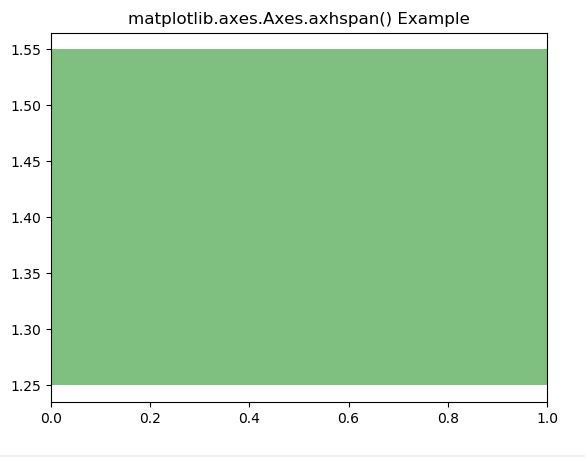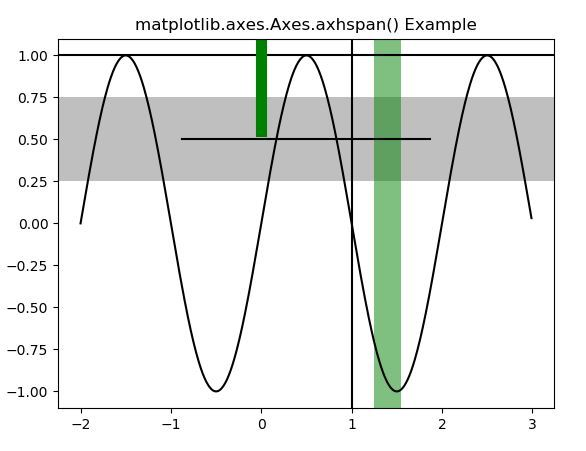# matplotlib.axes.Axes.axhspan() in Python

Matplotlib is a library in Python and it is numerical – mathematical extension for NumPy library. The Axes Class contains most of the figure elements: Axis, Tick, Line2D, Text, Polygon, etc., and sets the coordinate system. And the instances of Axes supports callbacks through a callbacks attribute.

## matplotlib.axes.Axes.axhspan() Function

The Axes.axhspan() function in axes module of matplotlib library is used to add a horizontal span (rectangle) across the axis.

Syntax:

`Axes.axhspan(self, ymin, ymax, xmin=0, xmax=1, **kwargs)`

Parameters: This method accept the following parameters that are described below:

• ymin: This parameter is the lower limit of the horizontal span in data units.
• ymax: This parameter is the upper limit of the horizontal span in data units.
• xmin: This parameter is the lower limit of the vertical span in data units.
• xmax: This parameter is the upper limit of the vertical span in data units.

Returns: This returns the Polygon.

Below examples illustrate the matplotlib.axes.Axes.axhspan() function in matplotlib.axes:

Example-1:

 `# Implementation of matplotlib function ` `import` `matplotlib.pyplot as plt ` `import` `numpy as np ` ` `  `fig, ax ``=` `plt.subplots() ` ` `  `ax.axhspan(``1.25``, ``1.55``, facecolor ``=``'g'``, alpha ``=` `0.5``) ` ` `  `ax.set_title(``'matplotlib.axes.Axes.axhspan() Example'``) ` ` `  `plt.show() `

Output:Example-2:

 `# Implementation of matplotlib function ` `import` `numpy as np ` `import` `matplotlib.pyplot as plt ` ` `  `t ``=` `np.arange(``-``2``, ``3``, .``01``) ` `s ``=` `np.sin(np.pi ``*` `t) ` ` `  `fig, ax ``=` `plt.subplots() ` `ax.plot(t, s, color ``=``'black'``) ` `ax.axhline(y ``=` `1``, color ``=``'black'``) ` `ax.axvline(x ``=` `1``, color ``=``'black'``) ` `ax.axvline(x ``=` `0``, ymin ``=` `0.75``, linewidth ``=` `8``, color ``=``'green'``) ` `ax.axhline(y ``=``.``5``, xmin ``=` `0.25``, xmax ``=` `0.75``, color ``=``'black'``) ` ` `  `ax.axhspan(``0.25``, ``0.75``, facecolor ``=``'0.5'``, alpha ``=` `0.5``) ` `ax.axvspan(``1.25``, ``1.55``, facecolor ``=``'green'``, alpha ``=` `0.5``) ` ` `  `ax.set_title(``'matplotlib.axes.Axes.axhspan() Example'``) ` ` `  `plt.show() `

Output:Attention geek! Strengthen your foundations with the Python Programming Foundation Course and learn the basics.

To begin with, your interview preparations Enhance your Data Structures concepts with the Python DS Course.

My Personal Notes arrow_drop_upSmall things always make you to think big

If you like GeeksforGeeks and would like to contribute, you can also write an article using contribute.geeksforgeeks.org or mail your article to contribute@geeksforgeeks.org. See your article appearing on the GeeksforGeeks main page and help other Geeks.

Please Improve this article if you find anything incorrect by clicking on the "Improve Article" button below.

Article Tags :

Be the First to upvote.

Please write to us at contribute@geeksforgeeks.org to report any issue with the above content.